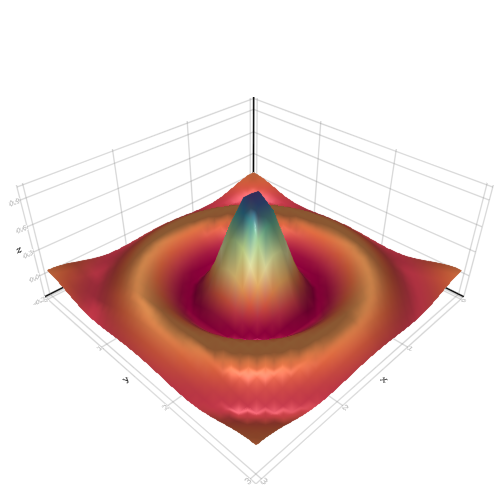## Test

```

using AbstractPlotting

NL = 30
NR = 31
function xy_data(x, y)
r = sqrt(x^2 + y^2)
r == 0.0 ? 1f0 : (sin(r)/r)
end
lspace = range(-10, stop = 10, length = NL)
rspace = range(-10, stop = 10, length = NR)

z = Float32[xy_data(x, y) for x in lspace, y in rspace]
l = range(0, stop = 3, length = NL)
r = range(0, stop = 3, length = NR)
surface(
[l for l in l, r in r], [r for l in l, r in r], z,
colormap = :Spectral
)

```